# Let’s do it…

## Step 1 — Importing libraries required for Fire and Smoke Detection.

`import osimport cv2import numpy as npimport matplotlib.pyplot as pltfrom keras.utils import np_utilsfrom tensorflow.keras.optimizers import SGDfrom sklearn.model_selection import train_test_splitfrom tensorflow.keras.models import Sequential,load_modelfrom tensorflow.keras.preprocessing.image import ImageDataGeneratorfrom tensorflow.keras.layers import BatchNormalization,Dense,SeparableConv2D,MaxPooling2D,Activation,Flatten,Dropout`

## Step 2 — Defining some constants.

`INIT_LR = 0.1BATCH_SIZE = 64NUM_EPOCHS = 50lr_find = Trueclasses = ['Non_Fire','Fire']`

## Step 3 — Reading images and storing them.

`images = []labels = []for c in classes:    try:        for img in os.listdir('Image Dataset/'+c):            img = cv2.imread('Image Dataset/'+c+'/'+img)            img = cv2.resize(img,(128,128))            images.append(img)            labels.append([0,1][c=='Fire'])    except:        pass    images = np.array(images,dtype='float32')/255.`
• Here we have just used cv2.imread() to read all the images and store them in an array.
• Also we are storing labels. 1 is for Fire and 0 for Non_Fire.
• In the last line we are just simply normalizing the images. Previously our images were form 0–255 but now they are from 0–1.

## Step 4 — Just randomly visualizing an image.

`ind = np.random.randint(0,len(images))cv2.imshow(str(labels[ind]),images[ind])cv2.waitKey(0)cv2.destroyAllWindows()`
• This image will be different every time because we have used random here.

## Step 5 — One hot encoding the labels.

`labels = np.array(labels)labels = np_utils.to_categorical(labels,num_classes=2)`

## Step 6 — Creating class weights dictionary.

`d = {}classTotals = labels.sum(axis=0)classWeight = classTotals.max() / classTotalsd = classWeightd = classWeight`
• Creating class weights dictionary to balance weights during training process.
• This process is done because our classes are not balanced in this case.
• Non_Fire images are double that of Fire Images.

## Step 7 — Train test splitting the data.

`X_train, X_test, y_train, y_test = train_test_split(images, labels, test_size=0.25, shuffle=True, random_state=42)`

## Step 8 — Initializing the data augmentation object.

`aug = ImageDataGenerator(    rotation_range=30,    zoom_range=0.15,    width_shift_range=0.2,    height_shift_range=0.2,    shear_range=0.15,    horizontal_flip=True,    fill_mode="nearest")`

## Step 9 — Creating the layout of the model.

`model = Sequential()# CONV => RELU => POOLmodel.add(SeparableConv2D(16,(7,7),padding='same',input_shape=(128,128,3)))model.add(Activation('relu'))model.add(BatchNormalization())model.add(MaxPooling2D(pool_size=(2,2)))# CONV => RELU => POOLmodel.add(SeparableConv2D(32,(3,3),padding='same'))model.add(Activation('relu'))model.add(BatchNormalization())model.add(MaxPooling2D(pool_size=(2,2)))# CONV => RELU => CONV => RELU => POOLmodel.add(SeparableConv2D(64,(3,3),padding='same'))model.add(Activation('relu'))model.add(BatchNormalization())model.add(SeparableConv2D(64,(3,3),padding='same'))model.add(Activation('relu'))model.add(BatchNormalization())model.add(MaxPooling2D(pool_size=(2,2)))# first set of FC => RELU layersmodel.add(Flatten())model.add(Dense(128))model.add(Activation('relu'))model.add(BatchNormalization())model.add(Dropout(0.5))# second set of FC => RELU layersmodel.add(Dense(128))model.add(Activation('relu'))model.add(BatchNormalization())model.add(Dropout(0.5))# softmax classifiermodel.add(Dense(len(classes)))model.add(Activation("softmax"))opt = SGD(learning_rate=INIT_LR, momentum=0.9,decay=INIT_LR / NUM_EPOCHS)model.compile(loss='binary_crossentropy',              optimizer=opt,              metrics=['accuracy'])print(model.summary())`

## Step 10 — Training and saving the model.

`print("[INFO] training network...")H = model.fit(    aug.flow(X_train, y_train, batch_size=BATCH_SIZE),    validation_data=(X_test, y_test),    steps_per_epoch=X_train.shape // BATCH_SIZE,    epochs=NUM_EPOCHS,    class_weight=d,    verbose=1)print("[INFO] serializing network to '{}'...".format('output/model'))model.save('output/fire_detection.h5')`
• Simply training and saving our model.
• The first line in model.fit is aug.flow(X_train, y_train, batch_size=BATCH_SIZE) which will create the augmented data.
• Also we have passed the class_weight parameter as d which we declared in previous steps.

## Step 11 — Visualizing the training process.

`N = np.arange(0, NUM_EPOCHS)plt.figure(figsize=(12,8))plt.subplot(121)plt.title("Losses")plt.plot(N, H.history["loss"], label="train_loss")plt.plot(N, H.history["val_loss"], label="val_loss")plt.subplot(122)plt.title("Accuracies")plt.plot(N, H.history["accuracy"], label="train_acc")plt.plot(N, H.history["val_accuracy"], label="val_acc")plt.legend()plt.savefig("output/training_plot.png")`

`# load the trained model from diskprint("[INFO] loading model...")model = load_model('output/fire_detection.h5')`

## Step 13 — Live prediction.

`for i in range(50):    random_index = np.random.randint(0,len(X_test))    org_img = X_test[random_index]*255    img = org_img.copy()    img = cv2.resize(img,(128,128))    img = img.astype('float32')/256    pred = model.predict(np.expand_dims(img,axis=0))    result = classes[np.argmax(pred)]    org_img = cv2.resize(org_img,(500,500))    cv2.putText(org_img, result, (35, 50), cv2.FONT_HERSHEY_SIMPLEX,1.25, (0, 255, 0), 3)    cv2.imwrite('output/testing/{}.png'.format(i),org_img)`

# Some examples…

--

--

--

## More from Abhishek Sharma

Data Scientist || Blogger || machinelearningprojects.net

Love podcasts or audiobooks? Learn on the go with our new app.

## Bringing Artificial Intelligence to the Edge with Offline First Web Apps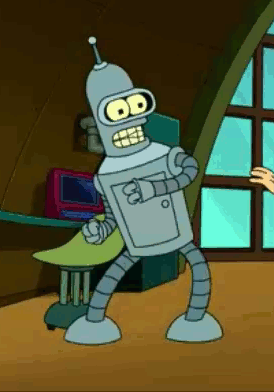## Automatic Log Analysis using Deep learning and AI — XenonStack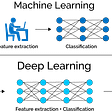## A Fair Comparison Study of XLNet and BERT with Large Models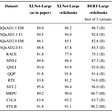## Machine Learning Basics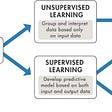## Machine Learning Use Cases in HealthCare that improve Patient Outcomes## Don’t be Afraid of Nonparametric Topic Models## Introduction to Genetic Algorithm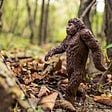## Abhishek Sharma

Data Scientist || Blogger || machinelearningprojects.net

## IMAGE CAPTIONING USING DEEP LEARNING — WITH SOURCE CODE — EASY EXPLANATION## Object Detection Using OpenCV## Detecting Melanoma Skin Cancer with Computer Vision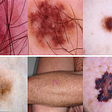## Text Detection With More Than 50 FPS on CPU : Python